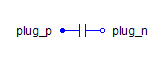Gauge Pressure Sensor - MapleSim Help

Pressure Sensor

Sense pressure at port with respect to gauge pressureDescription The Pressure Sensor component generates an output signal of the pressure at the port with respect to the gauge pressure.Equations $y=\mathrm{toUnit}\left({p}_{\mathrm{port}}-{P}_{0}\right)$ $q=0$Connections

 Name Description Modelica ID $\mathrm{port}$ Hydraulic port port $y$ Real output signal in selected units yParameters

 Name Default Units Description Modelica ID ${P}_{0}$ $0$ $\mathrm{Pa}$ Gauge pressure P0 To unit $\mathrm{Pa}$ Output unit toUnit